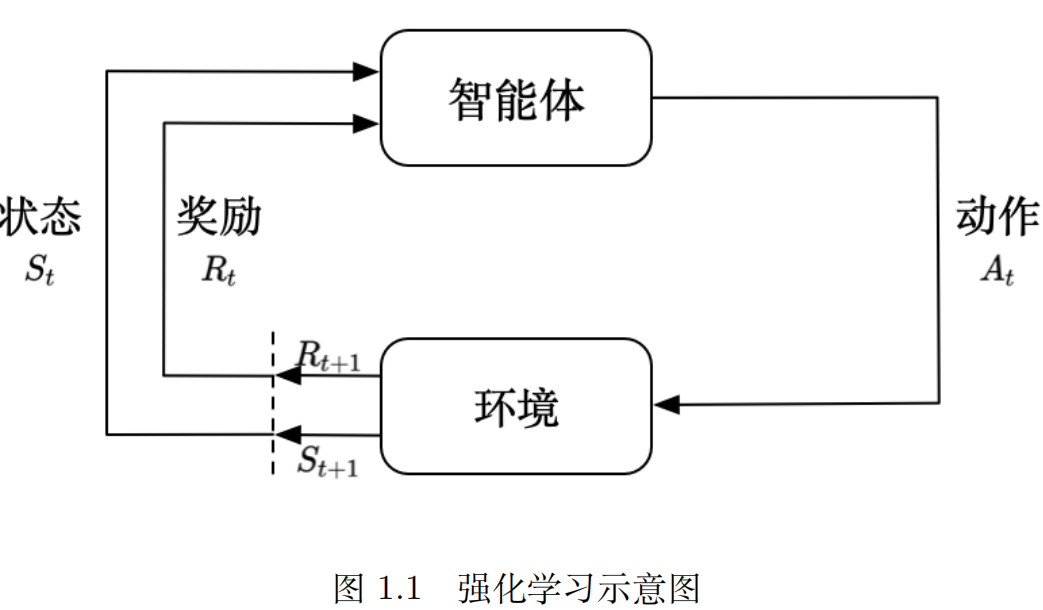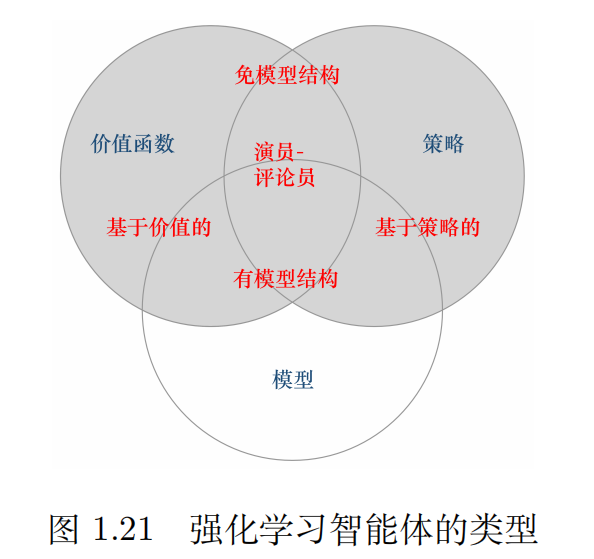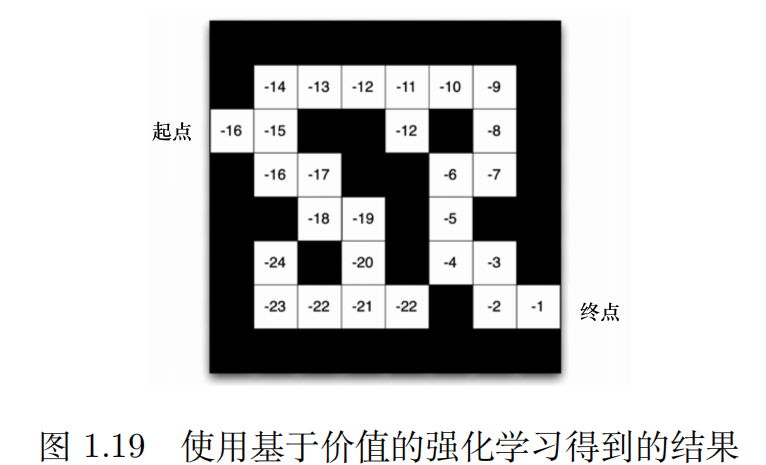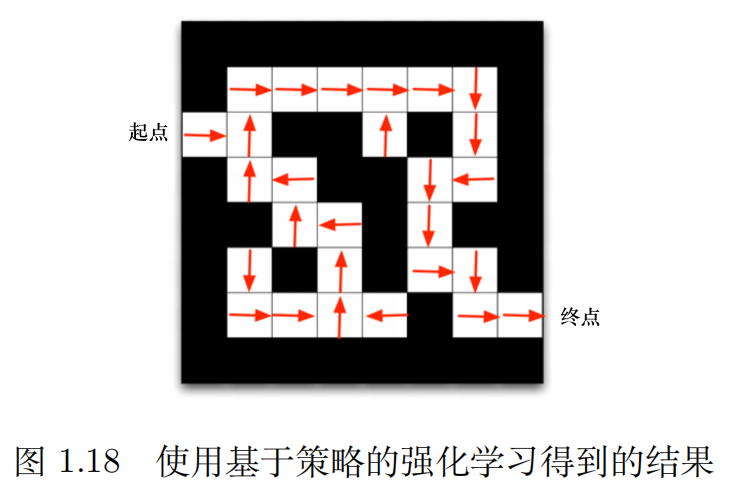## 1. 强化学习

（1）定义

（2）过程1: 智能体获取状态，并根据状态输出动作（决策）
2: 环境根据执行的决策输出下一状态和该决策获得的奖励

（3）强化学习与监督学习的比较
（1）强化学习输入的是序列数据，不满足独立同分布
（2）强化学习无法得到立即反馈
（3）延迟奖励（delayed reward）

（4）强化学习的分类
（1）标准强化学习：手动设置特征 + 强化学习
（2）深度强化学习： 深度强化学习 = 深度学习 + 强化学习。不需要手动设置特征，将学习过程改进为端到端的训练（end-to-end training）。可以用一个神经网络来拟合我们这里的价值函数或策略网络，省去了特征工程（featureengineering）的过程。

## 2. 序列决策（Sequential decision making）

（1）奖励

（2）序列决策

（3）状态

H t = O 1 , R 1 , A 1 , O 2 , ⋯   , O t , R t H_t=O_1,R_1,A_1,O_2,\cdots,O_t,R_t

S t = f ( H t ) S_t=f(H_t)

## 3. 动作空间

（1）离散动作空间（discrete action space）：智能体的动作数量是有限的
（2）连续动作空间（continuos action space）：在连续空间中，动作是实值的向量

## 4. 智能体的组成和类型

1. 策略函数（policy function）：把输入的状态变成行为
2. 价值函数（value function）：对当前状态进行评估（对后续收益的影响）
3. 模型（model）：表现智能体对环境的理解

### 4.1 策略

（1）定义

（2）分类

π \pi 函数 π ( a ∣ s ) = P ( A t = a ∣ S t = s ) \pi(a|s)=P(A_t=a|S_t=s) ，表示在状态s下输出动作为a的概率。然后通过采样得到一个动作。

#### 问题：比较随机性策略和确定性策略的优缺点

① 随机性能更好的探索环境
② 随机性策略的动作具有多样性（不是唯一确定的）
③ 确定性策略对相同环境做出相同的动作，这会导致很容易被预测

### 4.2 价值函数

（1）状态价值函数（state-value function）

v π ( s ) = E π [ G t ∣ S t = s ] = E π [ ∑ k = 0 ∞ γ k R t + k + 1 ∣ S t = s ]   , f o r   a l l   s ∈ S v_\pi(s)=E_\pi[G_t|S_t=s]=E_\pi[\sum_{k=0}^{\infty}\gamma^k R_{t+k+1}|S_t=s] \ , for \ all \ s \in S

（2）动作状态价值函数（state-action-value function）

q π ( s , a ) = E π [ G t ∣ S t = s , A t = a ] = E π [ ∑ k = 0 ∞ γ k R t + k + 1 ∣ S t = s , A t = a ] q_\pi(s,a)=E_\pi[G_t|S_t=s,A_t=a]=E_\pi[\sum_{k=0}^{\infty}\gamma^k R_{t+k+1}|S_t=s,A_t=a]

（3）Q函数和V函数的关系
v函数表示在状态s下，采取所有可能动作的未来奖励之和。
v π ( s ) = ∑ a ∈ A t q π ( s , a ) v_\pi(s)=\sum_{a \in A_t} q_\pi(s,a)

### 4.3 模型

（1）状态转移概率

P s s ′ a = P [ S t + 1 = s ′ ∣ S t = s , A t = a ] P_{ss'}^a=P[S_{t+1}=s'|S_t=s,A_t=a]

（2）奖励函数

R s a = E [ R t + 1 ∣ S t = s , A t = a ] R_s^a=E[R_{t+1}|S_t=s,A_t=a]

### 4.4 类型（1）基于价值的智能体（value-based agent）• Q-Learning
• Sarsa

（2）基于策略的智能体（policy-based agent）• 策略梯度算法

（3）有模型智能体（model-based agent）

（4）免模型智能体（model-free agent）

（1）学习（learning）

（2）规划（planning）

（3）解决思路

## 6. 探索和利用

（探索：看某个行为的奖励，利用：选取已知可以取得最好奖励的行为）

## Reference

https://github.com/datawhalechina/easy-rl